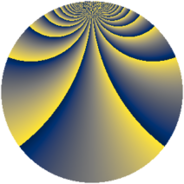# Properties

 Label 920.2.bnLevel $920$ Weight $2$ Character orbit 920.bn Rep. character $\chi_{920}(19,\cdot)$ Character field $\Q(\zeta_{22})$ Dimension $1400$ Newform subspaces $2$ Sturm bound $288$ Trace bound $1$

# Related objects

## Defining parameters

 Level: $$N$$ $$=$$ $$920 = 2^{3} \cdot 5 \cdot 23$$ Weight: $$k$$ $$=$$ $$2$$ Character orbit: $$[\chi]$$ $$=$$ 920.bn (of order $$22$$ and degree $$10$$) Character conductor: $$\operatorname{cond}(\chi)$$ $$=$$ $$920$$ Character field: $$\Q(\zeta_{22})$$ Newform subspaces: $$2$$ Sturm bound: $$288$$ Trace bound: $$1$$ Distinguishing $$T_p$$: $$3$$

## Dimensions

The following table gives the dimensions of various subspaces of $$M_{2}(920, [\chi])$$.

Total New Old
Modular forms 1480 1480 0
Cusp forms 1400 1400 0
Eisenstein series 80 80 0

## Trace form

 $$1400q - 22q^{4} - 30q^{6} + 96q^{9} + O(q^{10})$$ $$1400q - 22q^{4} - 30q^{6} + 96q^{9} - 11q^{10} - 44q^{11} - 22q^{14} - 14q^{16} - 44q^{19} - 11q^{20} - 48q^{24} - 18q^{25} - 30q^{26} - 11q^{30} - 44q^{34} - 38q^{35} + 2q^{36} - 11q^{40} - 36q^{41} + 110q^{44} - 14q^{46} + 80q^{49} - 15q^{50} - 44q^{51} - 34q^{54} - 22q^{56} - 36q^{59} - 11q^{60} - 58q^{64} - 22q^{65} - 88q^{66} - 14q^{70} - 22q^{74} - 30q^{75} - 132q^{76} + 88q^{80} - 152q^{81} - 22q^{84} - 242q^{86} - 44q^{89} + 220q^{90} - 110q^{94} - 456q^{96} - 44q^{99} + O(q^{100})$$

## Decomposition of $$S_{2}^{\mathrm{new}}(920, [\chi])$$ into newform subspaces

Label Dim. $$A$$ Field CM Traces $q$-expansion
$$a_2$$ $$a_3$$ $$a_5$$ $$a_7$$
920.2.bn.a $$40$$ $$7.346$$ $$\Q(\sqrt{-10})$$ $$0$$ $$0$$ $$0$$ $$0$$
920.2.bn.b $$1360$$ $$7.346$$ None $$0$$ $$0$$ $$0$$ $$0$$